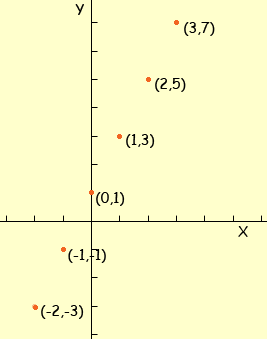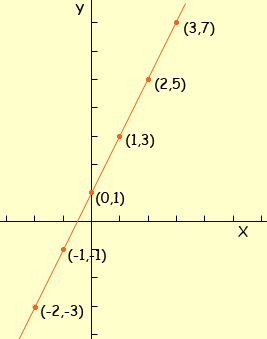Hi
My name is Geneva and I'm having trouble with graphing equations like y=2x+1 which is my homework I'm not sure how to graph it. I'm in the 8th grade taking a 9th grade course algebra I.
Thank you

Hi Geneva,

Sometimes, especially after a little experience, you can tell from the "form" of the equation what the "shape" of the graph is. If you can tell the shape then it makes plotting the graph easier, but for the moment let's just try to plot it without knowing the shape beforehand. To do this I am going to use a table of values. I am going to choose some values for the variable x, calculate the corresponding value of y and then record my results in a table. So, for example when x = 1 then y = 21 + 1 = 3 and when x = 2 then y = y = 22 + 1 = 5. Here is my table including these two values of x and a few more.

x y
1 3
2 5
3 7
0 1
-1 -1
-2 -3

Now I put these points on my graph.Now you can see the "shape" of the graph. If you have plotted these points accurately and you place a ruler along the points you will see that they all lie on a straight line.Graph of y = 2x + 1

If you knew at the beginning, from the "form" of the equation that the graph was a straight line then you could have plotted the graph more quickly. In this case you only need to calculate and plot two points and the line joining these two points is the graph.

I hope this helps,
Penny